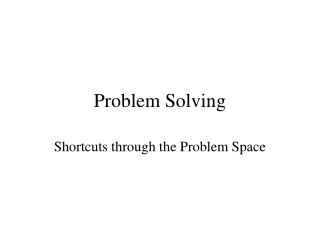DownloadDownload PresentationProblem Solving

# Problem Solving

Download Presentation## Problem Solving

- - - - - - - - - - - - - - - - - - - - - - - - - - - E N D - - - - - - - - - - - - - - - - - - - - - - - - - - -
##### Presentation Transcript

1. Problem Solving Shortcuts through the Problem Space

2. Problem Solving • Problem = a situation in which one is trying to reach a goal • Problem solving = finding a means for arriving at a goal

3. Stages of Problem Solving • Define the problem -- Problem identification and representation • Select a strategy -- plan a solution • Carry out the strategy -- execute the plan • Evaluate the plan and the solution -- determine whether it worked

4. Defining the Problem • Identify the initial state and the goal state • Well-defined Problem: clear definition of problem and goal states (example: locked out of your house) • Ill-defined Problem: the problem or goal state is not clearly defined (example: increase crop production in USSR -- Voss, Grene, Post, & Penner, 1983) • Decide how to represent the initial and goal states

5. Problem Representation • How difficult it is to solve a problem often depends on how you choose to represent it. • Some examples: • "number scrabble" (Newell & Simon, 1972) • "Bird and Trains" math problem (from Posner 1973, Cognition: An introduction) • The monk problem(Duncker, 1945)

6. Selecting a Strategy:Algorithms vs. Heuristics • Algorithm • a procedure that is guaranteed to produce a solution to the problem • Examples: • solving the anagram "xbo“ by enumerating all possible combinations: xbo, xob, oxb, obx, bxo, box • What about "ntraoc"? There are 6! (or 72) possible combinations.

7. Heuristics in Problem Solving • Heuristic = a rule of thumb, or "mental shortcut" for solving a problem • not guaranteed to give the right answer • usually much more efficient than an algorithm • Heuristics for solving anagrams: • “xbo” • vowel in the middle • assume “x” is not word-initial • “ntraoc” • start with likely groupings of letters: "ant, car, tan, tar, ton"

8. Problem Space and Computational Complexity • Problem space = all the possible states of affairs that could be produced from transformations of the initial problem state. • Problem solving consists of searching the problem space for a state that matches your goal state. • Algorithms search the entire space; heuristics search only part • If the problem space is too large, an algorithmic approach is impossible. Example: Chess.

9. Useful Problem-Solving Strategies (Heuristics) • Simple Search (Hill Climbing) • Means-end Analysis • Break the problem into subgoals • Used in the General Problem Solver (Newell & Simon, 1963; Newell, Simon, & Shaw, 1958) • Working Backwards. Useful when: • There is only one goal state and it is clearly specified • There are a number of possible ways to represent the problem state

10. Try Out Your Problem Solving Skills • The “Calvin & Hobbes” Game • The Water Jar Problem (Luchins, 1942) • The “9 Dots” Problem • Can you connect all 9 of these dots by drawing 4 straight lines, without lifting your pencil from the page? • Give up? Here is a solution.

11. “Set” in Problem Solving • "set" = state of mind a person brings to a problem solving situation • An inappropriate "set" can keep you from representing the problem in the most productive way, or from choosing the best solution strategy.

12. “Set” interfering with problem representation • The nine dots problem -- including an unnecessary boundary in your representation of the problem • Functional Fixedness: failing to see a new use for an object • Duncker (1945) -- mount a candle on the wall • The two string problem

13. “Set” interfering with choosing an effective solution strategy • "Persistence of set" in the water jar problem (Luchins, 1942)